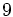# Group cohomology of symmetric group:S4

View group cohomology of particular groups | View other specific information about symmetric group:S4

## Classifying space and corresponding chain complex

The homology and cohomology groups are the same as the respective homology and cohomology groups of the configuration space of four unordered points in a countable-dimensional real projective space. For more on the topological perspective, see configuration space of unordered points of a countable-dimensional real vector space on the Topology Wiki.

## Family contexts

Family name Parameter value General discussion of group cohomology of family
symmetric group$S_n$ of degree$n$ degree$n = 4$ group cohomology of symmetric groups
projective general linear group of degree two$PGL(2,q)$ over a finite field of size$q$$q = 3$, i.e., field:F3 group cohomology of projective general linear group of degree two over a finite field

## Homology groups for trivial group action

FACTS TO CHECK AGAINST (homology group for trivial group action):
First homology group: first homology group for trivial group action equals tensor product with abelianization
Second homology group: formula for second homology group for trivial group action in terms of Schur multiplier and abelianization|Hopf's formula for Schur multiplier
General: universal coefficients theorem for group homology|homology group for trivial group action commutes with direct product in second coordinate|Kunneth formula for group homology

#### Over the integers

The homology groups over the integers have a description in terms of the congruence class of the homology degree modulo 4 as well as its quotient on integer division by 4.

The first few homology groups are given as follows:$q$$0$$1$$2$$3$$4$$5$$6$$7$$8$$9$$10$$H_q$$\mathbb{Z}$$\mathbb{Z}_2$$\mathbb{Z}_2$$\mathbb{Z}_2 \oplus \mathbb{Z}_4 \oplus \mathbb{Z}_3$$\mathbb{Z}_2$$(\mathbb{Z}_2)^3$$(\mathbb{Z}_2)^2$$(\mathbb{Z}_2)^2 \oplus \mathbb{Z}_4 \oplus \mathbb{Z}_3$$(\mathbb{Z}_2)^3$$(\mathbb{Z}_2)^4$ ($\mathbb{Z}_2)^3$

## GAP implementation

### Computation of homology groups

The homology groups for trivial group action on the integers can be computed using the Hap package (if the package is installed but not automatically loaded, load it using LoadPackage("hap");), specifically its GroupHomology function. The function returns a list of numbers which are the orders of cyclic groups whose external direct product is the desired homology group.

#### First homology group

The first homology group, which is also the abelianization, can be computed as follows:

```gap> GroupHomology(SymmetricGroup(4),1);
[ 2 ]```

This says that$H_1(S_4;\mathbb{Z}) = \mathbb{Z}/2\mathbb{Z}$.

#### Second homology group

The second homology group, which is also the Schur multiplier, can be computed as follows:

```gap> GroupHomology(SymmetricGroup(4),2);
[ 2 ]```

This says that$H_2(S_4;\mathbb{Z}) = \mathbb{Z}/2\mathbb{Z}$.

#### First few homology groups

```gap> List([1..10],i -> [i,GroupHomology(SymmetricGroup(4),i)]);
[ [ 1, [ 2 ] ], [ 2, [ 2 ] ], [ 3, [ 2, 4, 3 ] ], [ 4, [ 2 ] ],
[ 5, [ 2, 2, 2 ] ], [ 6, [ 2, 2 ] ], [ 7, [ 2, 2, 4, 3 ] ],
[ 8, [ 2, 2, 2 ] ], [ 9, [ 2, 2, 2, 2 ] ], [ 10, [ 2, 2, 2 ] ] ]```